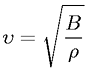Equations > Physics > Mechanics > Speed of sound waves in a fluid

### Speed of sound waves in a fluidLatex Code:

MathML Code:

 $\upsilon =\sqrt{\fracB\rho }$

MathType 5.0: